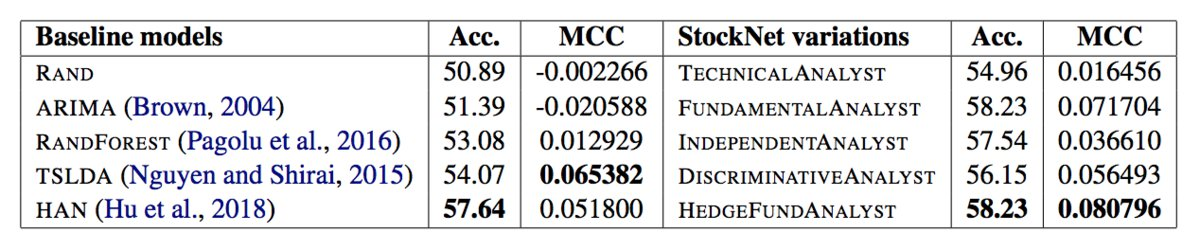# Stock Movement Prediction from Tweets and Historical Prices (Paper Summary)

This paper suggests a way of using both historical prices and text data together for financial time series prediction. They call it Stocknet. There seem to be 2 major contributions here: (a) Encoding both market data and text data together, (b) VAE (Variational AutoEncoder) inspired generative model.

## TLDR

RNN-based variational autoencoder along with attention is used to predict whether the stock price will go up or down.

## Dataset

• 88 stocks
• From 2014-01-01 to 2016-01-01. Training data range: 2014-01-01 to 2015-08-01 (20,339 samples). Validation: 2015-08-01 to 2015-10-01 (2555 datapoints). Testing: 2015-10-01 to 2016-01-01 (3720 datapoints).
• price_change <= -0.5% is assigned 0 label. price_change > 0.55% is assigned 1 label. The ones in between these 2 thresholds are ignored.

## Model

There are 3 main components here:

1. Market Information Encoder (MIE) - Encodes tweets and prices to X.
2. Variational Movement Decoder (VMD) - Infers Z with X, y and decodes stock movements y from X, Z.
3. Attentive Temporal Auxiliary (ATA) - Integrates temporal loss through an attention mechanism for model training.

### Market Information Encoder (MIE)

This component is relatively straightforward. Tweets for the given day are combined into the vector $$c_t$$. Historical prices are normalized and stored in the vector $$p_t$$. The output of this component (MIE) is the vector $$x_t = [c_t, p_t]$$.

### Variational Movement Decoder (VMD)

VMD uses the market information $$X$$ received from the previous component and infers a latent factor $$Z$$. This latent vector $$Z$$ is then decoded into vector $$y_t$$ using an RNN decoder with GRU cells.

### Attentive Temporal Auxiliary (ATA)

Attention is applied to the outputs from the previous component. Both VAE and Attention components are combined to construct the final loss function $$F$$.

$F(\theta, \phi, X, y) = \frac{1}{N}\sum_n^Nv^{(n)}f^{(n)}$

Here, $$v^{(n)}$$ is the attention weight vector and $$f^{(n)}$$ is the loss function from the variational autoencoder component.

\begin{aligned} f = \log p_{\theta} - \lambda D_{KL}[q_{\phi} \vert\vert p_{\theta}] \end{aligned}

$$\log p_{\theta}$$ is the log-likelihood term, $$D_{KL}[q_{\phi} \vert\vert p_{\theta}]$$ is the KL divergence loss and $$\lambda$$ is the KL loss weight. $$\lambda$$ is increased over time during training. It’s known as KL annealing trick Bowman et al., 2016.

## Training and Hyperparameters

• 5-day lag window is used to construct the dataset.
• Batch size is 32. Each batch contains randomly picked data points.
• Initial learning rate of Adam - 0.001
• Dropout rate - 0.3 for the hidden layer

## Metrics and Results

MCC (Matthews Correlation Coefficient) is used as a metric. MCC is defined below in terms of tp (true positives), tn (true negatives), fp (false positives) and fn (false negatives).

$MCC = \frac{tp \times tn - fp \times fn}{\sqrt{(tp + fp)(tp + fn)(tn + fp)(tn + fn)}}$Baselines:

TECHNICALANALYST, FUNDAMENTALANALYST, INDEPENDENTANALYST, DISCRIMINATIVEANALYST and HEDGEFUNDANALYST are simply different variants of their StockNet model with HEDGEFUNDANALYST being the model described above.